# Python爬虫初级案例——爬取网络小说

1、获取小说主页源代码

2、在主页源代码中找到每个章节的超链接

3、获取每个章节超链接的源代码

4、获取章节的内容

5、保存内容到本地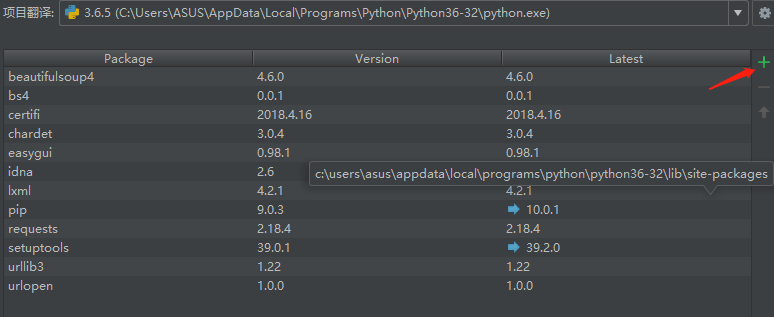```import re
import urllib.request```

```import re
import urllib.request

#定义一个爬取网络小说的函数
def getNovelContent():

```import re
import urllib.request

#定义一个爬取网络小说的函数
def getNovelContent():
html = html.decode("gbk")   #转成该网址的格式```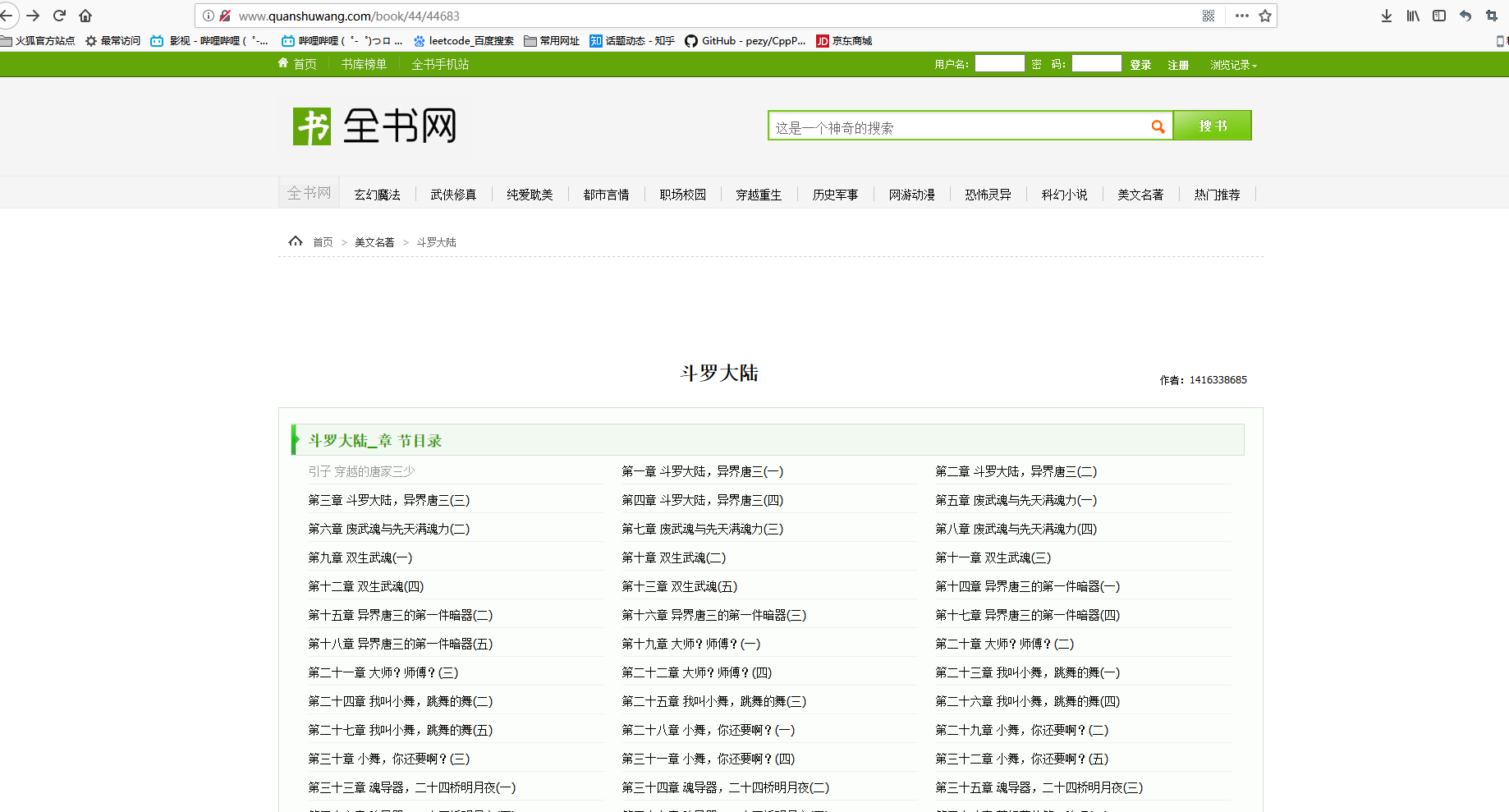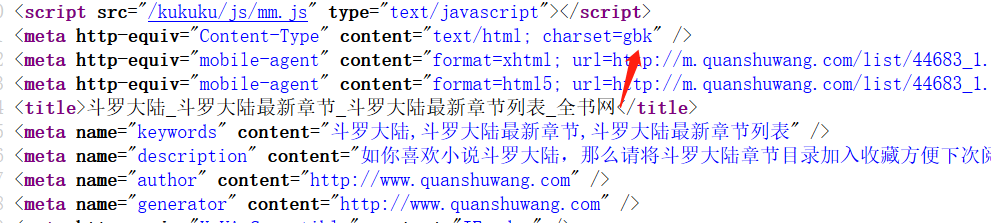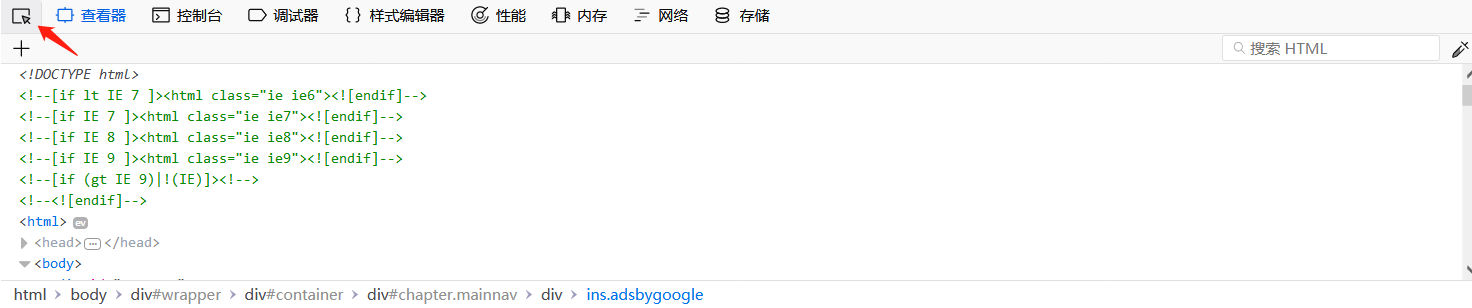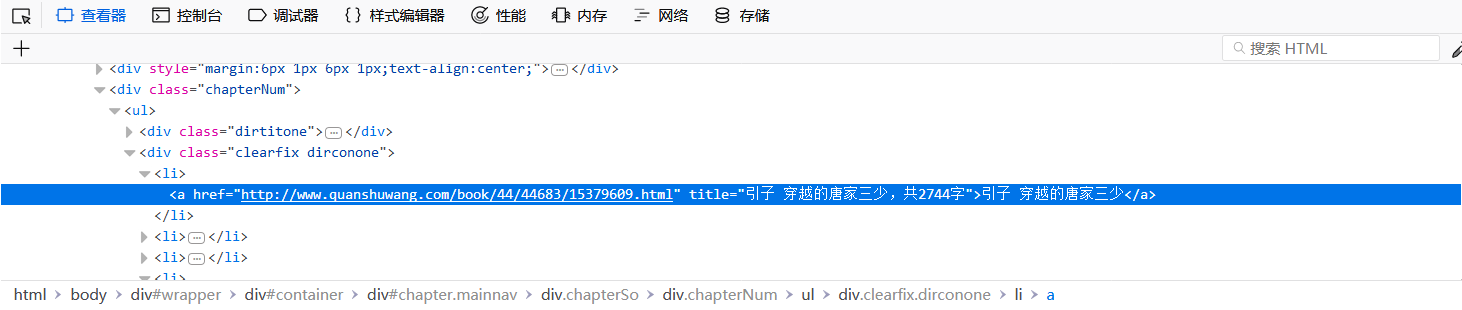```def getNovelContent():
html = html.decode("gbk")   #转成该网址的格式
#<li><a href="http://www.quanshuwang.com/book/44/44683/15379609.html" title="引子 穿越的唐家三少，共2744字">引子 穿越的唐家三少</a></li>  #参考```

```#定义一个爬取网络小说的函数
def getNovelContent():
html = html.decode("gbk")   #转成该网址的格式
#<li><a href="http://www.quanshuwang.com/book/44/44683/15379609.html" title="引子 穿越的唐家三少，共2744字">引子 穿越的唐家三少</a></li>  #参考
reg = r'<li><a href="(.*?)" title=".*?">(.*?)</a></li>'     #正则表达的匹配
reg = re.compile(reg)     #可添加可不添加，增加效率
urls = re.findall(reg,html)```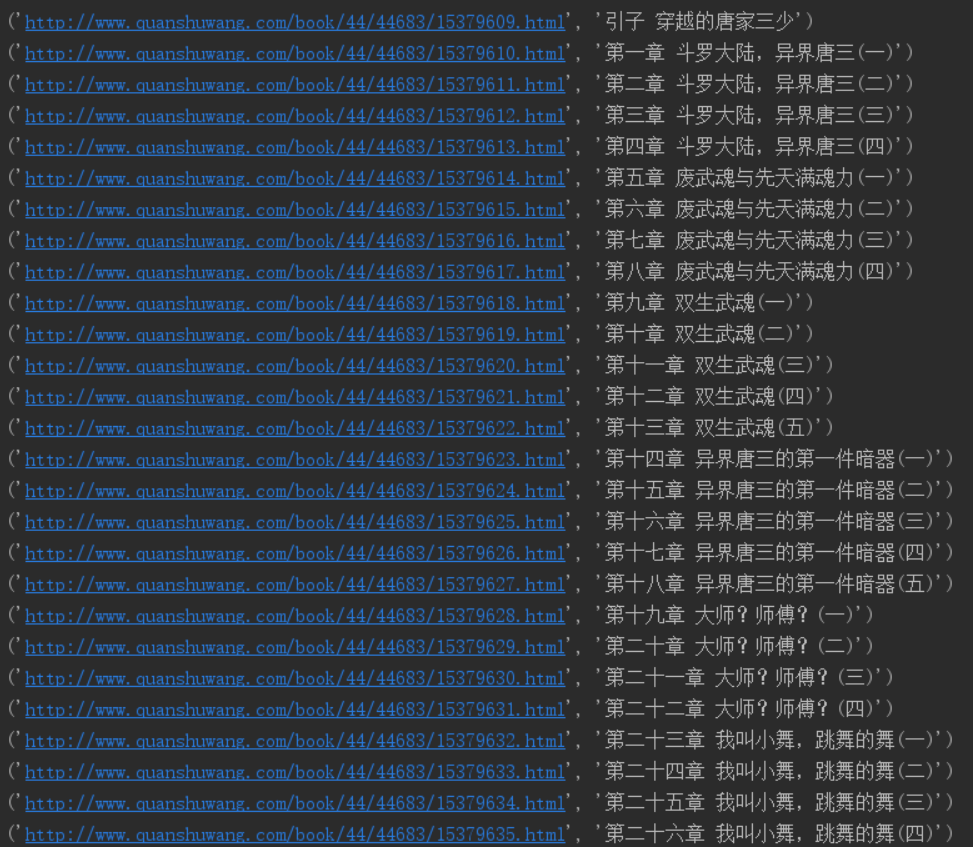```#定义一个爬取网络小说的函数
def getNovelContent():
html = html.decode("gbk")   #转成该网址的格式
#<li><a href="http://www.quanshuwang.com/book/44/44683/15379609.html" title="引子 穿越的唐家三少，共2744字">引子 穿越的唐家三少</a></li>  #参考
reg = r'<li><a href="(.*?)" title=".*?">(.*?)</a></li>'     #正则表达的匹配
reg = re.compile(reg)     #可添加可不添加，增加效率
urls = re.findall(reg,html)
for url in urls:
#print(url)
chapter_url = url  #章节的超链接
chapter_title = url  #章节的名字
print(chapter_title)
chapter_html = chapter_html.decode("gbk")```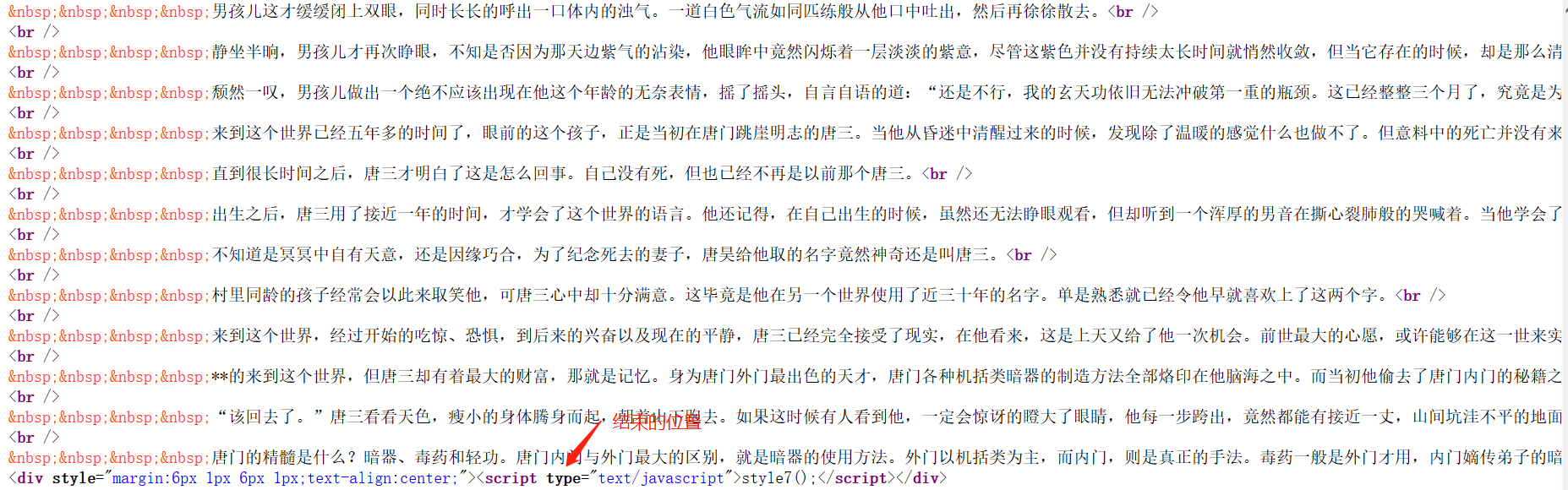```#定义一个爬取网络小说的函数
def getNovelContent():
html = html.decode("gbk")   #转成该网址的格式
#<li><a href="http://www.quanshuwang.com/book/44/44683/15379609.html" title="引子 穿越的唐家三少，共2744字">引子 穿越的唐家三少</a></li>  #参考
reg = r'<li><a href="(.*?)" title=".*?">(.*?)</a></li>'     #正则表达的匹配
reg = re.compile(reg)     #可添加可不添加，增加效率
urls = re.findall(reg,html)
for url in urls:
#print(url)
chapter_url = url  #章节的超链接
chapter_title = url  #章节的名字
print(chapter_title)
chapter_html = chapter_html.decode("gbk")
chapter_reg = r'</script>&nbsp;&nbsp;&nbsp;&nbsp;&nbsp;.*?<br />(.*?)<script type="text/javascript">'
chapter_reg = re.compile(chapter_reg,re.S)
chapter_content = re.findall(chapter_reg,chapter_html)```

#定义一个爬取网络小说的函数

```def getNovelContent():
html = html.decode("gbk")   #转成该网址的格式
#<li><a href="http://www.quanshuwang.com/book/44/44683/15379609.html" title="引子 穿越的唐家三少，共2744字">引子 穿越的唐家三少</a></li>  #参考
reg = r'<li><a href="(.*?)" title=".*?">(.*?)</a></li>'     #正则表达的匹配
reg = re.compile(reg)     #可添加可不添加，增加效率
urls = re.findall(reg,html)
for url in urls:
#print(url)
chapter_url = url  #章节的超链接
chapter_title = url  #章节的名字
print(chapter_title)
chapter_html = chapter_html.decode("gbk")
chapter_reg = r'</script>&nbsp;&nbsp;&nbsp;&nbsp;.*?<br />(.*?)<script type="text/javascript">'
chapter_reg = re.compile(chapter_reg,re.S)
chapter_content = re.findall(chapter_reg,chapter_html)
for content in chapter_content:                #打印章节的内容
content = content.replace("&nbsp;&nbsp;&nbsp;&nbsp","")  #把"&nbsp;"字符全都替换为""
content = content.replace("<br />","")      #把"<br/>"字符全部替换为""
print(content)                         #打印内容```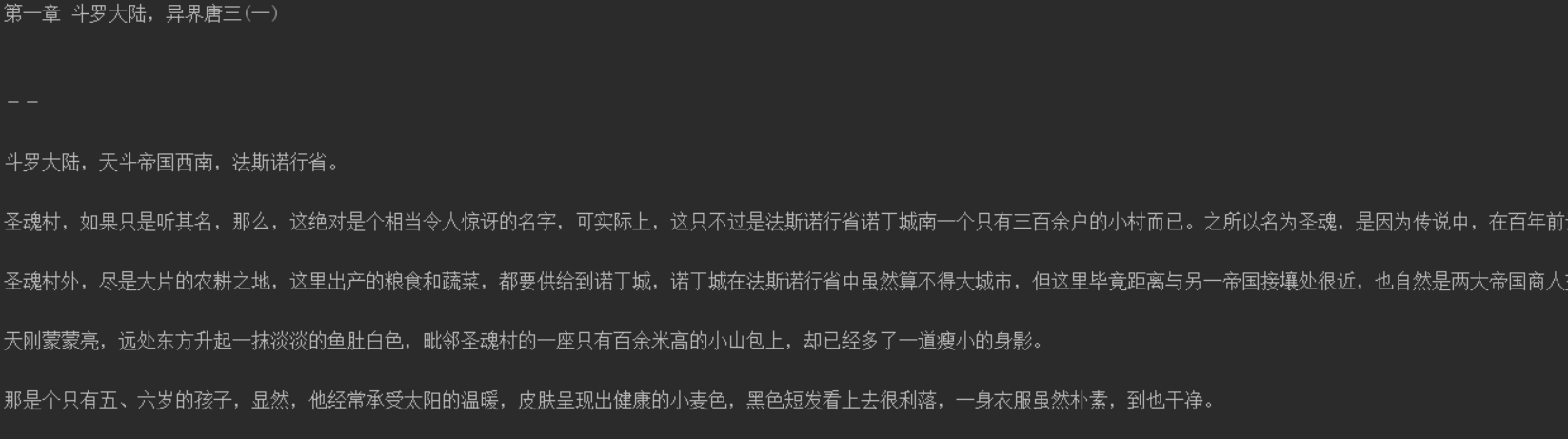#定义一个爬取网络小说的函数

```def getNovelContent():
html = html.decode("gbk")   #转成该网址的格式
#<li><a href="http://www.quanshuwang.com/book/44/44683/15379609.html" title="引子 穿越的唐家三少，共2744字">引子 穿越的唐家三少</a></li>  #参考
reg = r'<li><a href="(.*?)" title=".*?">(.*?)</a></li>'     #正则表达的匹配
reg = re.compile(reg)     #可添加可不添加，增加效率
urls = re.findall(reg,html)
for url in urls:
#print(url)
chapter_url = url  #章节的超链接
chapter_title = url  #章节的名字
print(chapter_title)
chapter_html = chapter_html.decode("gbk")
chapter_reg = r'</script>&nbsp;&nbsp;&nbsp;&nbsp;.*?<br />(.*?)<script type="text/javascript">'
chapter_reg = re.compile(chapter_reg,re.S)
chapter_content = re.findall(chapter_reg,chapter_html)
for content in chapter_content:
content = content.replace("&nbsp;&nbsp;&nbsp;&nbsp;","")
content = content.replace("<br />","")
print(content)
f = open('{}.txt'.format(chapter_title),'w')      #保存到文本
f.write(content)```

```import re
import urllib.request

#定义一个爬取网络小说的函数
def getNovelContent():
html = html.decode("gbk")   #转成该网址的格式
#<li><a href="http://www.quanshuwang.com/book/44/44683/15379609.html" title="引子 穿越的唐家三少，共2744字">引子 穿越的唐家三少</a></li>  #参考
reg = r'<li><a href="(.*?)" title=".*?">(.*?)</a></li>'     #正则表达的匹配
reg = re.compile(reg)     #可添加可不添加，增加效率
urls = re.findall(reg,html)
for url in urls:
#print(url)
chapter_url = url  #章节的超链接
chapter_title = url  #章节的名字
#print(chapter_title)
chapter_html = chapter_html.decode("gbk")
chapter_reg = r'</script>&nbsp;&nbsp;&nbsp;&nbsp;.*?<br />(.*?)<script type="text/javascript">'
chapter_reg = re.compile(chapter_reg,re.S)
chapter_content = re.findall(chapter_reg,chapter_html)
for content in chapter_content:
content = content.replace("&nbsp;&nbsp;&nbsp;&nbsp;","")
content = content.replace("<br />","")
# print(content)
f = open('{}.txt'.format(chapter_title),'w')
f.write(content)

getNovelContent()```本文由用户 【admin】 自行上传分享，仅供网友学习交流。所有权归原作者，若您的权利被侵害，请联系管理员。转载本站原创文章，请注明出处，并保留原始链接、图片水印。本站是一个以用户分享为主的开源技术平台，欢迎各类分享！本文地址：http://www.mj85.com/python/100.html分享：

### 评论 [共0条评论]

• 这篇文章还没有收到评论，赶紧来抢沙发吧~

19年/04月/30日 14时

Python爬虫

0人已收藏